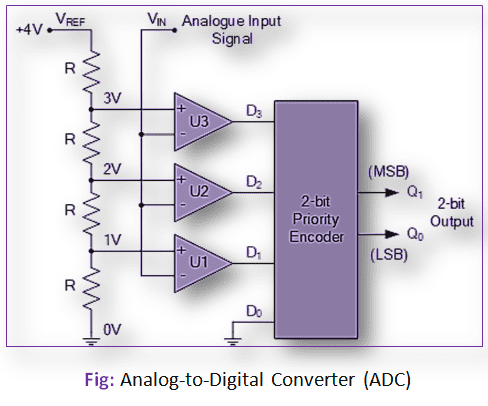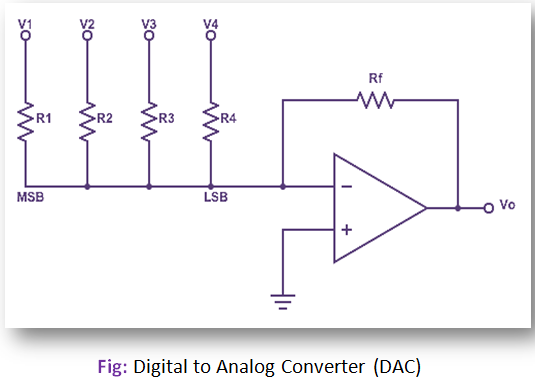# Difference between ADC and DAC

The Analog-to-Digital Converter (ADC) is the reverse process of Digital-to-Analog Converter (DAC). The ADC is also called quantization, in which the analog signal is represented by equivalent binary data. The analog signals vary continuously and are defined for any interval of time.The digital signals (or data) can take only finite values and are defined only for discrete instants of time. If the digital data is represented by n-bit binary then it can have 2n different values. In ADC, the given analog signal has to be divided into steps of 2n values, and each step is represented by one of the 2n values.

## DAC:

The Digital-to-Analog converter (DAC) is a process in which signals to have two defined levels or states are converted into signals having theoretically infinite number of states. The DAC will accept a digital (binary) input and convert it to analog voltage or current.